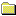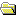Products Download Events Support Videos All Product Families ARM7, ARM9, and Cortex-M3 Products C16x, XC16x, and ST10 Products C251 and 80C251 Products Cx51 and 8051 Products Modified Anytime In the Last 7 Days In the Last 14 Days In the Last 30 Days In the Last 90 Days In the Last 6 Months

Technical Support

On-Line Manuals

CARM User's GuideCARM IntroductionCompiling ProgramsLanguage ExtensionsPreprocessorAdvanced ProgrammingCustomization FilesStartup CodeBasic I/OMemory AllocationInterfacing C to AssemblerFunction ParametersFunction Return ValuesUsing the SRC DirectiveRegister UsageExample RoutinesARM Code ExampleThumb Code ExampleData Storage FormatsByte OrderByte AlignmentInteger Scalars1-Byte Scalars2-Byte Scalars4-Byte Scalars8-Byte Scalarsfloat Scalarsfloat Formatfloat Errorsdouble Scalarsdouble Formatdouble ErrorsPointersBit FieldsAbsolute Memory AccessThe __at KeywordLinker Location ControlsError MessagesLibrary ReferenceAppendix

# <!-- var kmNextPage = "ca_ap_float_errors.htm"; var kmPrevPage = "ca_ap_float.htm"; var kmNextPageTitle = "float Errors"; var kmPrevPageTitle = "float Scalars"; var kmBreadCrumbs = "<a href=\"default.htm\">Home</a> &raquo; <a href=\"ca_advanced.htm\">Advanced Programming</a> &raquo; <a href=\"ca_ap_datastorage.htm\">Data Storage Formats</a> &raquo; <a href=\"ca_ap_float.htm\">float Scalars</a> &raquo; float Format"; kmSetupPaging (); kmNavButtons(); //--> float Format

A floating-point number is expressed as the product of three parts: the sign, the mantissa, and an exponent. For example:

```float value = sign × 1.mantissa × 2exponent-bias
```

Where

 sign Represents the sign of the floating-pointer number (+ or -). mantissa Represents the actual binary digits of the floating-point number. It is a 24-bit value (representing about seven decimal digits) whose most significant bit (MSB) is always 1 and is, therefore, not stored. exponent Is an 8-bit value from 0 to 255. The actual value of the exponent is calculated by subtracting the bias (127) from the stored value (0 to 255) giving a range of –127 to +128. bias Is a constant value (127) subtracted from the stored exponent to obtain the actual exponent.

Floating-point numbers are stored in normalized form which maximizes the quantity of numbers that can be represented. Normalized numbers have a binary point ('.') after the first non-zero digit. This is how the mantissa is able to hold 24 binary digits in only 23 bits.

Denormalized floating-point numbers are used to represent values smaller than what can be represented by normalized values. The drawback is that the precision decreases with smaller values. Denormalized floating-point values are represented as follows:

```float value = sign × 0.mantissa × 2-126
```

Where

 sign Represents the sign of the floating-pointer number (+ or -). mantissa Represents the actual binary digits of the floating-point number. It is a 24-bit value whose most significant bit (MSB) is always 0 and is not stored. -126 Is the fixed value of the exponent.

If the stored exponent is zero and the mantissa is non-zero the floating-point value is a denormalized number. For denormalized numbers, the exponent is treated as if a 1 were stored. Hence, the actual exponent is -126 (1 minus 127).

Floating-point numbers are stored using the following 32-bit format:

Bits 31-24 23-16 15-8 7-0
Contents `SEEE EEEE` `EMMM MMMM` `MMMM MMMM` `MMMM MMMM`

Where

 S represents the sign bit where 1 is negative and 0 is positive. E is the exponent with a bias of 127. M is the 24-bit mantissa (stored in 23 bits).

Using the above format, the floating-point number -12.5 is stored as a hexadecimal value of 0xC1480000. In memory, this value appears as follows:

Bits 31-24 23-16 15-8 7-0
Contents 0xC1 0x48 0x00 0x00

It is fairly simple to convert floating-point numbers to and from their hexadecimal storage equivalents. The following example demonstrates how this is done for the value -12.5 shown above.

The floating-point storage representation is not an intuitive format. To convert this to a floating-point number, the bits must be separated as specified in the floating-point number storage format table shown above. For example:

Bits 31-24 23-16 15-8 7-0
Format `SEEE EEEE` `EMMM MMMM` `MMMM MMMM` `MMMM MMMM`
Binary `1100 0001` `0100 1000` `0000 0000` `0000 0000`
Hex `C 1` `4 8` `0 0` `0 0`

From this illustration, you can determine the following:

• The sign bit is 1, indicating a negative number.
• The exponent value is 10000010 binary or 130 decimal. Subtracting 127 from 130 leaves 3, which is the actual exponent.
• The mantissa appears as the following binary number:
```10010000000000000000000
```

There is an understood binary point at the left of the mantissa that is always preceded by a 1. This digit is omitted from the stored form of the floating-point number. Adding 1 and the binary point to the beginning of the mantissa gives the following value:

```1.10010000000000000000000
```

To adjust the mantissa for the exponent, move the decimal point to the left for negative exponent values or right for positive exponent values. Since the exponent is three, the mantissa is adjusted as follows:

```1100.10000000000000000000
```

The result is a binary floating-point number. Binary digits to the left of the decimal point represent the power of two corresponding to their position. For example, 1100 represents (1 × 23) + (1 × 22) + (0 × 21) + (0 × 20), which is 12.

Binary digits to the right of the decimal point also represent the power of two corresponding to their position. However, the powers are negative. For example, .100... represents (1 × 2-1) + (0 × 2-2) + (0 × 2-3) + ... which equals .5.

The sum of these values is 12.5. Because the sign bit was set, this number should be negative.

So, the hexadecimal value 0xC1480000 is -12.5.##### Important information

This site uses cookies to store information on your computer. By continuing to use our site, you consent to our cookies.